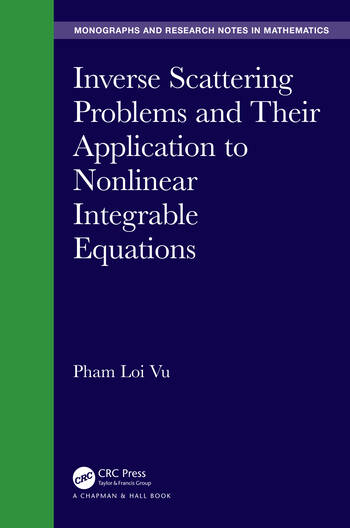# Inverse Scattering Problems and Their Application to Nonlinear Integrable Equations

## 1st Edition

Pham Loi Vu

Chapman and Hall/CRC
October 10, 2019 Forthcoming
Reference - 416 Pages
ISBN 9780367334895 - CAT# 317688
Series: Chapman & Hall/CRC Monographs and Research Notes in Mathematics

USD\$149.95

Availabe for Pre-Order. This item will ship after October 10, 2019
FREE Standard Shipping!

## Preview

### Summary

Inverse Scattering Problems and Their Application to Nonlinear Integrable Equations is devoted to inverse scattering problems (ISPs) for differential equations and their application to nonlinear evolution equations (NLEEs). The book is suitable for anyone who has a mathematical background and interest in functional analysis, partial differential equations, equations of mathematical physics, and functions of a complex variable. This book is intended for a wide community working with inverse scattering problems and their applications; in particular, there is a traditional community in mathematical physics.

In this monograph, the problems are solved step-by-step, and detailed proofs are given for the problems to make the topics more accessible for students who are approaching them for the first time.

Features

• The unique solvability of ISPs are proved. The scattering data of the considered inverse scattering problems (ISPs) are described completely.
• Solving the associated IVP or IBVP for the nonlinear evolution equations (NLEEs) is carried out step-by-step. Namely, the NLEE can be written as the compatibility condition of two linear equations. The unknown boundary values (BVs) are calculated, then the time-dependent scattering data (SD) are constructed from the given initial and boundary conditions.
• The potentials are recovered uniquely in terms of time-dependent SD, and the solution of the NLEEs is expressed uniquely in terms of the found solutions of the ISP.
• Since the considered ISPs are solved well, then the SPs generated by two linear equations constitute the ISM. The application of ISM to solving the NLEEs is consistent and is effectively embedded in the schema of the ISM.